•研究论文
• 该项目旨在彻底理解ICCV2017上的论文“使用相机响应模型的新型微光图像增强算法”的实现细节。 原始项目由Matlab实施。 由于项目是通过python复制的，因此某些关键功能无法正确复制。 如果您对本项目有帮助，并有...
• 高动态范围成像技术能够避免因为拍摄方向如...所提出的算法通过单帧输入图像获得成像系统的相机响应函数，大大地提高了计算效率，并且适用于欠曝光及过曝光条件下采集的图像，扩展了相机响应函数标定算法的应用范围。
• 基于某型号大气辐射测量卫星在轨定标实验数据，开展了空间污染物对天基红外测量相机响应性能的影响研究。建立了具有明确物理意义的测量相机灰度响应衰减模型，使用实测数据对模型参数进行了拟合，拟合相对均方根误差...
• 针对基于边缘流和传统ISODATA（迭代自组织的数据分析算法）相融合的算法对砾岩图像进行分割时存在速度慢、分割不准确的问题，提出了一种融合Canny和改进ISODATA聚类相结合的砾岩图像分割算法。该算法将图像从RGB色彩...
• 一、相机成像过程 拍摄对象反射回来的...这是电子成像部分，亦即相机响应过程。 二、相机响应函数 相机响应函数（CRF）就是传感器上曝光度III与图像亮度值MMM之间的映射关系： 也就是这个曲线图 横坐标：真实亮度

一、相机成像过程

拍摄对象反射回来的光线（场景辐射度）通过相机的镜头转换为传感器辐射度，传感器辐射度通过快门的通电作用转化为传感器曝光度。这是光学变化过程。传感器上的感光器件受到光照激发释放出电荷产生电信号。电信号经过放大和滤波后再进行模数转换得到数字信号，即图像数据。这些数据经过信号处理器进行相应的处理后存储为图像文件得以输出。这是电子成像部分，亦即相机响应过程。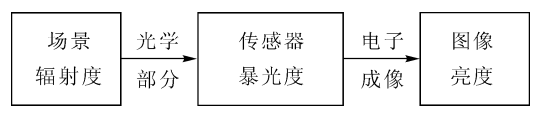二、相机响应函数

相机响应函数（CRF）就是传感器上曝光度 I I 与图像亮度值 M M 之间的映射关系：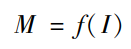也就是这个曲线图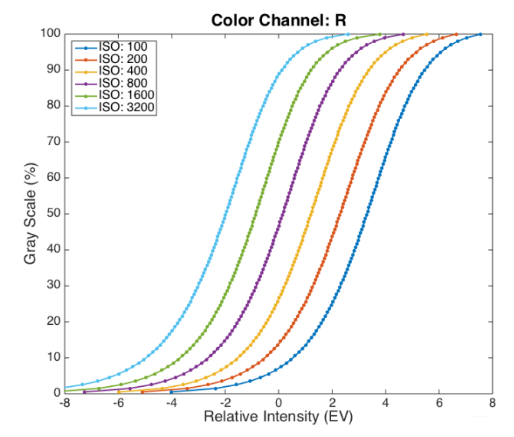横坐标：真实亮度
纵坐标：像素亮度
ISO：感光度，底片对光的灵敏程度。当ISO高时，就算真实亮度很小，所成像的像素亮度也会很大，整体会变亮。

三、相机响应逆函数

已知 M = f ( I ) M = f(I) ，那么如果将对 f f 求逆函数就会得到原来的曝光度：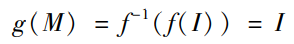此时 g ( M ) g(M) 与曝光度 I I 是线性关系。

展开全文• Debevec方法求相机响应曲线

千次阅读 2020-04-07 18:58:25
（3） 求出相机响应函数之后便可将图像的像素值转化为场景中的照度值: ln⁡Ei=g(Zij)−ln⁡Δtj \ln E_{i}=g(Z_{ij})-\ln \Delta t_{j} lnEi​=g(Zij​)−lnΔtj​ 由于图像中对应场景的同一个像素点都得到了多次...

该方法由Debevec在1997年的论文《Recovering High Dynamic Range Radiance Maps from Photographs》中提出。原文及翻译可以参考博客《Recovering High Dynamic Range Radiance Maps from Photographs》

变量约定

X X ：曝光量（the exposure），单位： J m − 2 Jm^{-2}
Δ t \Delta t ：曝光时间（the exposure time）；
X X E E Δ t \Delta t 的关系： X = E Δ t X=E\Delta t

Z Z ：数字图像的像素值；
f f ：相机的响应函数（Camera response function）；
Z Z X X f f 的关系： Z = f ( X ) Z=f(X)

算法

对同一场景使用不同的曝光时间进行拍摄，得到 P P 张图像，每一张的曝光时间记为 Δ t j \Delta t_{j} 1 ≤ j ≤ P 1\leq j\leq P 。每张图片上都取相同位置的 N N 个点， i i 为像素点的索引， 1 ≤ i ≤ N 1\leq i\leq N

假设场景是静态的，并且曝光过程完成得足够快，可以安全地忽略灯光的变化。然后可以假设像素 i i 的辐照度值 E i E_{i} 是常数。

Z i j Z_{ij} 表示第 j j 张图片的第 i i 个像素的值，则有
Z i j = f ( E i Δ t j ) Z_{ij}=f(E_{i}\Delta t_{j})
假设函数 f f 单调可逆，则有
f − 1 ( Z i j ) = E i Δ t j f^{-1}(Z_{ij})=E_{i}\Delta t_{j}
对上式两边取对数，则有
ln ⁡ f − 1 ( Z i j ) = ln ⁡ E i + ln ⁡ Δ t j \ln f^{-1}(Z_{ij})=\ln E_{i}+\ln \Delta t_{j}
为了方便表示，将 ln ⁡ f − 1 \ln f^{-1} 表示为 g g ，则可以得到方程组
g ( Z i j ) = ln ⁡ E i + ln ⁡ Δ t j g(Z_{ij})=\ln E_{i}+\ln \Delta t_{j}
在这个方程组中， Z i j Z_{ij} Δ t j \Delta t_{j} 是已知的。未知数是辐照度 E i E_{i} ，还有函数 g g

我们注意到，恢复函数 g g 只需要恢复 g ( z ) g(z) 可以取的有限数量的值，因为 Z Z 的域，即像素亮度值，是有限的。假设 Z m i n Z_{min} Z m a x Z_{max} 是最小和最大像素值（整数），那么，我们可以将问题表示为找到 ( Z m a x − Z m i n + 1 ) (Z_{max} - Z_{min} + 1) g ( Z ) g(Z) 值和 N N ln ⁡ E i \ln E_{i} 值。由于 1 ≤ i ≤ N 1\leq i\leq N 1 ≤ j ≤ P 1\leq j\leq P ，所以方程的个数为 N P NP 。我们可以使得 N P ≥ Z m a x − Z m i n + 1 + N NP\geq Z_{max} - Z_{min} + 1+N ，即方程个数大于未知数个数，构成一个超定方程组，可以用最小二乘法求取。使得目标函数
O = ∑ i = 1 N ∑ j = 1 P [ g ( Z i j ) − ln ⁡ E i − ln ⁡ Δ t j ] 2 （ 1 ） O=\sum_{i=1}^{N}\sum_{j=1}^{P}[g(Z_{ij})-\ln E_{i}-\ln \Delta t_{j}]^{2} \qquad（1）
的值为最小的未知数的值就是该方程组的最小二乘解。又假定相机响应函数是一条光滑曲线，Debevec 在上式中添加一项，使解出来的值能够成光滑曲线。
O = ∑ i = 1 N ∑ j = 1 P [ g ( Z i j ) − ln ⁡ E i − ln ⁡ Δ t j ] 2 + λ ∑ Z = Z m i n + 1 Z m a x − 1 g ′ ′ ( z ) 2 （ 2 ） O=\sum_{i=1}^{N}\sum_{j=1}^{P}[g(Z_{ij})-\ln E_{i}-\ln \Delta t_{j}]^{2}+\lambda\sum_{Z=Z_{min}+1}^{Z_{max}-1}g^{''}(z)^{2}\qquad（2）
上式中的 g ′ ′ ( z ) g^{''}(z) g ( z ) g(z) 的二阶导数。由于 g ( z ) g(z) 为离散函数， g ′ ′ ( z ) g^{''}(z) 定义如下：
g ′ ′ ( z ) = g ( z − 1 ) − 2 g ( z ) + g ( z + 1 ) g^{''}(z)=g(z-1)-2g(z)+g(z+1)
原文说可以用奇异值分解（SVD）对上述方程（2）进行求解。

另外，通过预测响应函数的基本形状，可以使求解具有更好的拟合性。由于 g ( z ) g(z) 通常在 Z m i n Z_{min} Z m a x Z_{max} 附近有一个陡峭的斜率，所以我们应该预计在这些极端附近， g ( z ) g(z) 将不太平滑并且更不拟合数据。为了认识到这一点，我们可以引入加权函数 w ( z ) w(z) 来强调曲线中间的平滑度和拟合项。函数 w w 的一个明智的选择是简单的hat函数：
w ( z ) = { z − Z m i n f o r   z ≤ 1 2 ( Z m i n + Z m a x ) Z m a x − z f o r   z > 1 2 ( Z m i n + Z m a x ) w(z)=\begin{cases} z-Z_{min}\quad for\ z\leq \frac{1}{2}(Z_{min}+Z_{max}) \\ Z_{max}-z\quad for\ z> \frac{1}{2}(Z_{min}+Z_{max}) \end{cases}
则方程（2）变为
O = ∑ i = 1 N ∑ j = 1 P { w ( Z i j ) [ g ( Z i j ) − ln ⁡ E i − ln ⁡ Δ t j ] } 2 + λ ∑ Z = Z m i n + 1 Z m a x − 1 [ w ( z ) g ′ ′ ( z ) ] 2   。 （ 3 ） O=\sum_{i=1}^{N}\sum_{j=1}^{P}\{w(Z_{ij})[g(Z_{ij})-\ln E_{i}-\ln \Delta t_{j}]\}^{2}+\lambda\sum_{Z=Z_{min}+1}^{Z_{max}-1}[w(z)g^{''}(z)]^{2}\ 。\qquad（3）

求出相机响应函数之后便可将图像的像素值转化为场景中的照度值:
ln ⁡ E i = g ( Z i j ) − ln ⁡ Δ t j \ln E_{i}=g(Z_{ij})-\ln \Delta t_{j}
由于图像中对应场景的同一个像素点都得到了多次曝光，为了加强鲁棒性，在恢复场景照度时应该使用所有曝光值所对应的照度，则每个像素点对应的照度是所有值的加权平均：
ln ⁡ E i = ∑ j = 1 P w ( Z i j ) [ g ( Z i j ) − ln ⁡ Δ t j ] ∑ j = 1 P w ( Z i j ) （ 4 ） \ln E_{i}=\frac{\sum_{j=1}^{P}w(Z_{ij})[g(Z_{ij})-\ln \Delta t_{j}]}{\sum_{j=1}^{P}w(Z_{ij})}\quad（4）
w ( z ) w(z) 是一个三角形的权重函数，当像素值为 0 或 255 时对应的权重为 0，像素值越靠近中间权重越大。这是因为越靠近 0 或 255 的像素值欠曝或过曝的可能性越高，可信度越低，对应的权重也越小。由式 （4）可从不同曝光的图像中重建高动范围态图像。

参考：
1.博客《Recovering High Dynamic Range Radiance Maps from Photographs》.
2.张东阳. 基于FPGA的高动态范围成像技术研究[D]. 华南理工大学, 2015.

展开全文算法
• I=f(V(t⋅L))I=f(V( t\cdot L))I=f(V(t⋅L)) 其中III为相机灰度值Intensity，LLL为环境中irradiance，ttt为曝光时间，VVV为相机镜头参数，fff为相机响应函数CRF。 有些论文中认为相机镜头参数是线性的不考虑，但有些...

本文主要用于记录自己在学习CRF过程中遇到的一些问题。

两个论文截图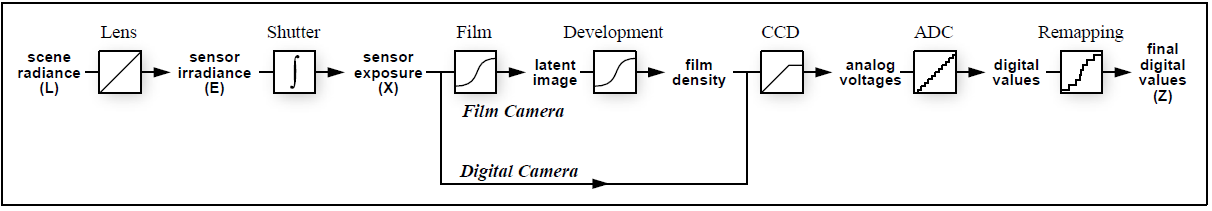CRF模型

B = f ( V ( t ⋅ L ) ) B=f(V( t\cdot L)) 其中 B B 为相机灰度值brightness， L L 为环境中radiance， t t 为曝光时间， V V 为相机镜头参数， f f 为相机响应函数CRF

有些论文中认为相机镜头参数是线性的不考虑，但有些考虑了衰减即靠近图像边缘亮度会暗一些，但没有看到二者同时计算的，如果同时计算两个非线性的函数，我觉得可能会有不确定性，在这里还请教一下了解的朋友。

控制相机曝光

首先要确定其中曝光时长 t t 。由于采用的是V4L2的USB相机，调整过程中遇到了一些列问题。详细记录在这里：V4L相机使用记录

利用OpenCV获取HDR图像

首先了解如何用多张图合成HDR图像，主要参考：learnopencv网站

伪代码：

// load images & exposure time
images.push_back(src);
const vector<float> times = {1/30.0f, 0.25, 2.5, 15.0};

// 计算CRF
Mat responseDebevec;  // response if a CV_32FC3 value.
Ptr<CalibrateDebevec> calibrateDebevec = createCalibrateDebevec();
calibrateDebevec->process(images, responseDebevec, times);

// 采用Debevec方法计算HDR
Mat hdr;
Ptr<MergeDebevec> mergeDebevec = createMergeDebevec();
mergeDebevec->process(images, hdr, times, responseDebevec);
// map to ldr for draw/showing.
Mat ldr;
Ptr<Tonemap> tonemap = createTonemap(2.2f);		// Debevec算法需要经过一步色调映射
tonemap->process(hdr, ldr);

// 采用Mertens方法计算HDR（不需要已知曝光参数）
Ptr<MergeMertens> mergeMertens = createMergeMertens();		// Mertens算法不要
Mat mm_img;
mergeMertens->process(images, mm_img);

注意：所有数据格式采用float或32FC1/32FC3格式，这个坑了我半天。

计算CRF

上面出现的responseDebevec就是CRF。这里把尝试把它画出来：

const int width_scale = 3, height_scale = 20;
const int width = 255 * width_scale, height = 40 * height_scale;
Mat img = Mat::zeros(Size(width, height), CV_8UC3);
Point b_old = Point(0, height);
Point g_old = Point(0, height);
Point r_old = Point(0, height);
for (int i = 0; i < 255; ++i){
Vec3f v3f = responseDebevec.at<Vec3f>(i, 0);
float ib = v3f;
float ig = v3f;
float ir = v3f;
Point b_new = Point(i * width_scale, height - ib * height_scale);
Point g_new = Point(i * width_scale, height - ig * height_scale);
Point r_new = Point(i * width_scale, height - ir * height_scale);
line(img, b_old, b_new, Scalar(255, 0, 0));
line(img, g_old, g_new, Scalar(0, 255, 0));
line(img, r_old, r_new, Scalar(0, 0, 255));
b_old = b_new;
g_old = g_new;
r_old = r_new;
}
imshow("crf", img);但遇到了一个问题，就是我用相机拍摄不同图像时，计算出的CRF是不一样的。趋势可能接近，但数值相差很多。我也不知道为啥，猜测可能和opencv通过V4L设置曝光时间时存在问题？但大致上都符合exp指数形式，那就认为差不都吧。

由于我每次测得的CRF不一样，但基本符合指数形式，故采用： B = a ⋅ e x p ( b ⋅ L ⋅ t ) + c B=a\cdot exp(b\cdot L \cdot t)+c 方式推算。这个方程也是我自己瞎凑的，给定 B = 0 , L = 0 B=0, L=0 B = 255 , L = 1 B=255, L=1 两个边界，假设 a = 1 a=1 ，计算反推：

inline double calInverseCRF(uchar intensity){
// Model equation: a x exp(b x intensity) - 1 = irradiance;
const float maxIrradiance = 1;       // normalized to 1.
const float a = 1.0f;                // given a value;
const float b = (log(maxIrradiance + 1) - log(a)) / 255;
return a * exp(b * intensity) - 1;
}

Mat testImg;
cvtColor(images, testImg, COLOR_BGR2GRAY);
assert(testImg.type() == CV_8UC1);
imshow("test", testImg);
for(int i=0; i<testImg.rows; ++i){
for(int j=0; j<testImg.cols; ++j){
float value = testImg.at<uchar>(i, j);
}
}
const double exposureTime = times;                                                // not used now.
Mat intensity = irradiance / exposureTime;
normalize(intensity, intensity, 1.0, 0.0, NORM_MINMAX);
imshow("dst", intensity);

结果：遇到的问题总结

1. CRF是三通道的，我尝试转成灰度图算一个CRF，计算的总是错误。
展开全文opencv
• 相机响应曲线、ISO详解

千次阅读 2018-11-16 17:14:17
相机响应曲线：外界光对应到拍摄的图像的像素值，中间的转化过程用曲线表示。 ISO：感光度，衡量底片对光的灵敏程度。 上面这幅图就是相机的响应曲线 横坐标为真实亮度的对数 纵坐标为像素亮度/底片密度——在这里...

相机响应曲线：外界光对应到拍摄的图像的像素值，中间的转化过程用曲线表示。
ISO：感光度，衡量底片对光的灵敏程度。上面这幅图就是相机的响应曲线
横坐标为真实亮度的对数
纵坐标为像素亮度/底片密度——在这里我们一般使用8bit的图，也就是（0,255），因此当灰度级别在50时，我们图像的值为0.5X255=78；灰度级别在100时，我们的图像的值为1X255=255
我们可以看出，响应是一条s曲线，s曲线的好处就是较低数值响应不敏感，较高数值响应不敏感，中间数值响应敏感。人眼对中间灰度敏感，因此s曲线刚好提高了中间灰度的辨识度，又不至于直接丢失暗部和亮部的信息。
这里我们还是需要用到输入、输出分析
输入：场景中，物体表面光的亮度
输出：拍摄图片中，该物体反映到数字（0-255）的灰度值。
中间过程：输出=响应曲线X（输入）——I=f(j)

我们这张图同样可以看出我们的ISO做了哪些工作。数码相机的ISO是通过调整感光元件对光线的灵敏程度或者合并感光点来实现的对相机相应曲线进行调节，这里的调节一般情况下只是在x轴方向上的平移。
ISO增大带来的影响是图像整体变亮了，实质为暗部向灰部迁移，灰部向亮部迁移，亮部全部过爆255。在图中我们能够看到，ISO变大，相机响应曲线左移，因此相机的暗部变为了敏感区域，而亮部则变成了100%的区域。
这里就可以说明了，ISO变化只起到调节相机响应曲线左右移的左右，而不会改变相机响应曲线。

展开全文相机响应曲线 相机响应 ISO
• 相机的灰度值与实际辐照度值的关系不成线性关系，因此需要进行预标定。 2.算法（Debeve and Malik） 图片引自知乎文章，横坐标表示曝光度ln（E*t），因为辐照度E不知道，所以假设为1，纵坐标表示像素值灰度百分比...图像处理
• 新的相机响应曲线函数标定算法，运用了最小二乘法和B样条曲线拟合，能有助于HDR的合成相机响应曲线
• https://blog.csdn.net/weixin_37970359/article/details/105361886 https://blog.csdn.net/u013049912/article/details/85157402 PDF:https://sci-hub.tw/10.1145/258734.258884
• 开发了一种改进的光谱反射率估计方法，以将原始相机的RGB响应转换为光谱反射率。 我们方法的新颖之处在于将局部加权线性回归模型应用于光谱反射率估计，并在CIELAB均匀色彩空间中使用高斯函数构造加权矩阵。 使用...研究论文
•研究论文
• 在多光源下基于CIE XYZ三刺激值从相机响应估计光谱反射率研究论文
• 请问有哪位大神做过基于单幅图像的边缘色彩信息求相机响应（逆）函数的matlab仿真，指点一二啊！跪谢！相机响应函数 辐射定标 边缘色彩信息
• 基恩士照相机

2019-01-30 11:31:31
基恩士摄像头操作手册，自带触摸屏，可在触摸屏上操作
• 当辐照光强较低时, CCD相机输出图像中的灰度值分布代表了辐照光的功率密度分布。利用此时CCD相机输出激光光斑的灰度分布及辐照激光功率计算了光斑中心像素上的平均激光功率密度。利用激光功率密度与激光功率成正比例...
• 数码相机通常具有线性曝光响应，但是噪声量和动态范围会有所不同。 很难获得有关这些参数的定量信息以进行明智的评估。 这项工作探索并演示了测量曝光响应的第一性原理，以便在不同相机型号之间进行有意义的比较。 ...
• 从理论上分析了中子在CCD相机拍摄图像中引起的瞬态干扰噪声的机理,并针对...研究得出了噪声特征随入射中子注量变化的规律,比较了不同能量及不同入射角条件下的噪声特性,并分析了两种不同结构的CCD相机响应特性的差别。
• 工业相机基本参数及选型

千次阅读 2021-04-20 16:46:56
点击上方“小白学视觉”，选择加"星标"或“置顶”重磅干货，第一时间送达 本文转自|新机器视觉 工业相机基本参数及选型工业相机是机器视觉系统中的一个关键组件，其最基础功能就...人工智能 计算机视觉 编程语言 opencv python
• 按照传感器的结构特性可以分为线阵相机（黑白摄像机、3Line彩色相机、3CCD彩色相机（分光棱镜）、面阵相机（黑白摄像机、Bayer彩色相机、3CCD彩色相机（分光棱镜）； 按照输出信号方式可以分为模拟相机（PAL（黑白...计算机视觉 工业相机
• 相机每次采集图像的像素点数，一般对应于光电传感器靶面排列的像元数，如1920*1080。 像素深度 每位像素数据的位数，常见的是8bit，10bit，12bit。分辨率和像素深度共同决定了图像的大小。例如对于像素深度为8...
• 相机处理后，读出时间1000 / 15 = 66.6666 毫秒(15为最大帧率) 使用千兆网连接，其理论最大速度125MB/s，按理想状态传递 ((3686400/1024/1024) / 125 )*1000= 28.12 毫秒 总耗费时间合计： 66.67 + 28.12 = 94
•  FLIR红外相机十分贵重，使用的时候轻拿轻放，供电务必注意：电源是标准的5v(可最大带600mA),由于内部没有过压保护电路，大于6v会烧毁相机；双光吊舱或单光吊舱之类的产品，会经常使用这种相机！ 1.相机调试--app-...红外相机...